Online Tution   »   Class 10 Science   »   Decomposition Reaction Examples

# Decomposition Reaction, Examples, Formula, Types, Definition For Class 10

Decomposition reactions are the breakdown of chemical species into simpler components. Typically, decomposition reactions necessitate the addition of energy. The breakdown of potassium chlorate (KClO3) by heat, for example, is a typical way of creating oxygen gas in the laboratory.

## Decomposition Reaction Definition

Decomposition reactions are a type of chemical reaction in which a single compound is broken down into two or more simpler compounds or elements. These reactions typically involve the breaking of chemical bonds, and they can be either endothermic or exothermic.

## Decomposition Reaction Example

One common example of a decomposition reaction is the breakdown of water into hydrogen and oxygen through the process of electrolysis. Another example is the thermal decomposition of calcium carbonate into calcium oxide and carbon dioxide.

## Decomposition Reaction Types

There are two main types of decomposition reactions: thermal decomposition and electrolytic decomposition.

1. Thermal Decomposition: Thermal decomposition occurs when a compound is broken down by heat. This type of decomposition is often exothermic, meaning it releases heat energy. Thermal decomposition is often used in the production of metals and other materials.
2. Electrolytic Decomposition: Electrolytic decomposition occurs when an electric current is passed through a compound, causing it to break down into simpler compounds or elements. This type of decomposition is often endothermic, meaning it requires the input of heat energy. It is used in the electrolysis of water to produce hydrogen and oxygen.

In general, a decomposition reaction can be represented by the general equation:

AB → A + B

where A and B represent the simpler products formed from the original compound AB.

Decomposition reactions are important in various industrial processes, such as the production of fertilizers, the extraction of metals, and the production of chemicals. The decomposition of compounds can also be used for purification and analysis of substances.

It’s important to note that Decomposition reactions can also be catalyzed by various agents such as light, catalysts, and enzymes.

## Decomposition Reaction Formula

 2KClO3(s) → 2KCl(s) + 3O2(g)

Another breakdown reaction is the electrolysis of molten sodium chloride (NaCl) at high temperatures to produce sodium (Na) and chlorine (Cl2).

 2NaCl (l) → 2Na (l) + Cl2(g)

The decomposition of mercury oxide (HgO) with heat to give mercury metal (Hg) and oxygen gas is a very important decomposition process in the history of chemistry. Carl Wilhelm Scheele, Joseph Priestley, and Antoine-Laurent Lavoisier employed this reaction in their investigations on oxygen in the 18th century.

 2HgO(s) → 2Hg (l) + O2(g)

## Decomposition Reaction- Format for General Reactions

A decomposition reaction’s general format is shown below.

AB -> A + B

The parent molecule (reactant) is AB, while the resultant molecules are A and B.

## Decomposition Reaction Examples for Class 10

Decomposition reactions occur all around us, although we are generally unaware of them. The following are some common instances of decomposition reactions.

The chemical equation

 H2CO3 → H2O + CO2

can be used to depict the decomposition of carbonic acid in soft drinks.

Water is electrolyzed to produce hydrogen and oxygen.

## Decomposition Reaction- Inverse Reaction

A combination reaction is the polar opposite of a decomposition process. The creation of a single product from two or more reactants is the goal of these reactions.

## Decomposition Reactions- Applications

The extraction of metals from their ores is one of the most common uses of decomposition processes. Zinc, for example, can be extracted from calamine by a decomposition reaction. Sodium can also be created from sodium chloride in a similar way (NaCl).

## Decomposition Reaction- Reaction of Double Decomposition

A double decomposition reaction occurs when two constituent reactants exchange positive and negative ions, resulting in the formation of two new compounds.

Example

 HCl(aq) + NaOH(aq) → NaCl(aq) + HOH(l)

## Examples of Decomposition Reaction- Types

The following are the three main types of breakdown reactions:

### Decomposition Reaction of thermal degradation

A decomposition reaction that is activated by thermal energy is known as a thermal decomposition reaction. To put it another way, a thermal decomposition reaction necessitates the delivery of energy to the reactants in the form of heat. Because energy is required to break chemical bonds and separate the constituent elements, such reactions are usually endothermic. The following is an example of a thermal breakdown reaction.

 CaCO3 → CaO + CO2

Calcium carbonate decomposes into calcium oxide and carbon dioxide when heated. This procedure is used to make quick lime, which is a critical ingredient in a variety of industries.

### Decomposition Reaction of electrolytic decomposition

The activation energy for decomposition in an electrolytic decomposition reaction is delivered in the form of electrical energy. The electrolysis of water is an example of an electrolytic breakdown reaction, which can be represented by the chemical equation:

 2H2O→ 2H2 + O2

### Photo decomposition/ Photolytic breakdown/ Photochemical decomposition

A photodecomposition reaction is a sort of decomposition reaction in which the reaction absorbs energy from photons and breaks down into its parts. The decomposition of dioxygen and an oxygen radical, as indicated by the chemical equation below, is an example of a photo decomposition reaction.

 O3 + hv → O2 + O

Related Post:

## 10 Examples of Decomposition Reaction

1. The decomposition of hydrogen peroxide (H2O2) into water (H2O) and oxygen (O2) is an example of a decomposition reaction: 2H2O2 –> 2H2O + O2
2. The decomposition of water into hydrogen and oxygen through the process of electrolysis is another example of a decomposition reaction: 2H2O –> 2H2 + O2
3. The decomposition of ammonium nitrate (NH4NO3) into nitrogen gas (N2) and water vapor (H2O) is another example: NH4NO3 –> N2 + H2O
4. The decomposition of calcium carbonate (CaCO3) into calcium oxide (CaO) and carbon dioxide (CO2) also represents a decomposition reaction: CaCO3 –> CaO + CO2
5. The decomposition of potassium chlorate (KClO3) into potassium chloride (KCl) and oxygen (O2) is another example: KClO3 –> KCl + 3O2
6. The decomposition of potassium permanganate (KMnO4) into manganese dioxide (MnO2) and oxygen (O2) is another example: 2KMnO4 –> 2KMnO2 + O2
7. The decomposition of sodium bicarbonate (NaHCO3) into sodium carbonate (Na2CO3), water (H2O) and carbon dioxide (CO2) also represents a decomposition reaction: NaHCO3 –> Na2CO3 + H2O + CO2
8. The decomposition of sulfuric acid (H2SO4) into sulfur dioxide (SO2) and water (H2O) is another example: 2H2SO4 –> 2SO2 + 2H2O
9. The decomposition of hydrogen sulfide (H2S) into sulfur (S) and water (H2O) is another example: H2S –> S + H2O
10. The decomposition of nitric oxide (NO) into nitrogen gas (N2) and oxygen (O2) is another example: 2NO –> N2 + O2

These are just a few examples of decomposition reactions, but there are many more that occur in nature and in various industrial processes.

Sharing is caring!

## FAQs

### What are 2 examples of decomposition reactions?

When one reactant breaks down into two or more products, it is called a decomposition reaction. AB A + B is a general equation that can be used to represent this. The breakdown of hydrogen peroxide to water and oxygen, as well as the breakdown of water to hydrogen and oxygen, are examples of decomposition reactions.

### Which is decomposition reaction?

Decomposition reactions are the breakdown of chemical species into simpler components. Typically, decomposition reactions necessitate the addition of energy. The breakdown of potassium chlorate (KClO3) by heat, for example, is a typical way of creating oxygen gas in the laboratory.

### What are the types of decomposition reactions with examples?

A photodecomposition reaction is a sort of decomposition reaction in which the reactant absorbs energy from photons and breaks down into its parts. The decomposition of dioxygen and an oxygen radical, as indicated by the chemical equation below, is an example of a photo decomposition reaction.

### What are the 5 types of chemical reactions?

The five types of chemical reactions are: Combination (Synthesis) reaction. (A + B → AB), Decomposition reaction. (AB → A + B), Displacement reaction. (A + BC → AB + C), Double displacement reaction. (AB + CD → AD + BC), and Combustion reaction.

### Give Examples of Decomposition Chemical Reactions.

Every day examples of Decomposition reaction in our lives are:
• Electrolysis: Water ---> hydrogen and water
• When a soft drink loses its bubbles: Carbonic acid ---> Carbon dioxide and water
• Digestion process: Food ---> proteins and carbohydrates
• Silver chloride ---> silver and chlorine.

### TOPICS:

•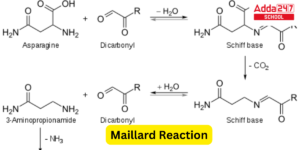Maillard Reaction Temperature, Mechanism...
•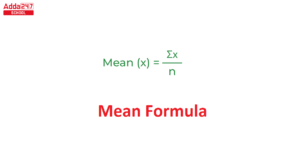Mean Formula and Meaning in Maths
•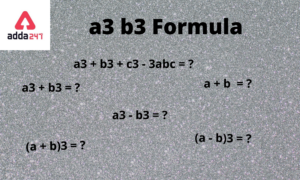a3 b3 Formula- Proof, Solution and Examp...
•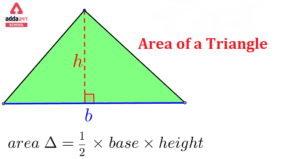Area of Triangle- Formula in Class 10 Co...
•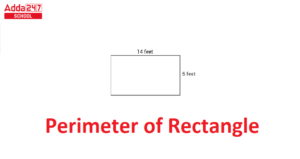Perimeter of Rectangle Formula Unit, Que...
•Compound Interest Formula, Definition, C...